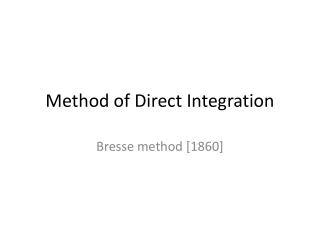# Method of Direct Integration - PowerPoint PPT PresentationDownload PresentationMethod of Direct Integration

Method of Direct IntegrationDownload Presentation## Method of Direct Integration

- - - - - - - - - - - - - - - - - - - - - - - - - - - E N D - - - - - - - - - - - - - - - - - - - - - - - - - - -
##### Presentation Transcript

1. Method of Direct Integration Bresse method 

2. Bresse Method • We have seen that for a wide rectangular channel and • This means , (or approx. ), and • Using the same equations introduced at (Chow, 1959) method we can say that: Where can be found from the varied-flow function table. • Mathematical integration of can be estimated as: (Bresse integration)

3. Bresse Method • The critical and normal depths in a wide rectangular channel may be expressed as: , ( is Chezy coefficient)

4. Bresse Method • Then, where , and Therefore the length of flow profile between two consecutive sections of depths is

5. Example • A trapezoidal channel having , , , and , carries a discharge of . Compute the backwater profile created by a dam which backs up the water to a depth of immediately behind the dam. The upstream end of profile is assumed at a depth equal to greater than the normal depth. The energy coefficient